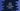# Java Program to check if a number is Neon or not## Java Program to check if a number is Neon number or not:

In this Java tutorial, we will learn how to check if a number is ’Neon’ number or not. A ’Neon’ number is a number whose sum of all digits of square of the number is equal to the number. For example, ‘9’ is a Neon number. Because, square of 9 is 9*9= 81. Sum of all digits of 81 is 8+1=9. So it is a Neon number. Similarly 1 is also a Neon number. But 8 is not.

Algorithm we are going to use is :

### Algorithm to check if a number is Neon or not :

1. Use one while loop. This loop will exit only if user enters -1 as input number. Otherwise, get user input and check if it is ‘Neon’ or not for infinite time.
2. First take the input number from user.
3. Calculate the square of the number.
4. Now, find the_ sum of all the digits of the square number_ using a loop.
5. Finally, check if the sum is equal to the given number or not.
6. If equal, it is a Neon number. Else it is not.
``````import java.util.Scanner;

public class Main {

/**
* Utility function for System.out.println
*
* @param message : string to print
*/
private static void println(String message) {
System.out.println(message);
}

/**
* Method to check if a number is Neon or not
*
* @param n : Number to check
* @return : true if 'n' is a neon number, false otherwise
*/
private static boolean isNeonNumber(int n) {
int square = n * n;

int sum = 0;

//find the sum of all digits of square
while (square > 0) {
sum += square % 10;
square = square / 10;
}

//return true if the sum is equal to the input number
return (sum == n);
}

/**
* main method
*
* @throws java.lang.Exception
*/
public static void main(String[] args) throws java.lang.Exception {
Scanner sc = new Scanner(System.in);
int no;

while (true) {
println("");
println("Enter a number to check if it is Neon or not. ( -1 to exit ) : ");
no = sc.nextInt();

if (no == -1) {
break;
}

if (isNeonNumber(no)) {
println("Input no is Neon.");
} else {
println("Input no is not Neon.");
}
}

}

}``````

### Sample Output :

``````Enter a number to check if it is Neon or not. ( -1 to exit ) :
12
Input no is not Neon.

Enter a number to check if it is Neon or not. ( -1 to exit ) :
9
Input no is Neon.

Enter a number to check if it is Neon or not. ( -1 to exit ) :
90
Input no is not Neon.

Enter a number to check if it is Neon or not. ( -1 to exit ) :
-1

Process finished with exit code 0``````